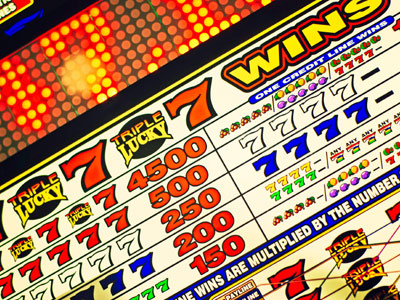Test your math knowledge in this quiz.

# Numbers (Classification)

This Math quiz is called 'Numbers (Classification)' and it has been written by teachers to help you if you are studying the subject at middle school. Playing educational quizzes is a fabulous way to learn if you are in the 6th, 7th or 8th grade - aged 11 to 14.

It costs only \$12.50 per month to play this quiz and over 3,500 others that help you with your school work. You can subscribe on the page at Join Us

A number is a number, right? The trouble is, there are so many numbers, and different properties that they can have, that we need some way of grouping, or classifying, them. We first learn about numbers as a small child, counting up from 1. These are the ‘counting’ numbers, more properly called NATURAL numbers. Then the concept of zero is introduced, so 0, 1, 2, 3 etcetera become the WHOLE numbers.

As we develop, we become aware of the concept of negative numbers, numbers that start at zero on the number line but go the other way. Whole numbers that can be positive or negative are called INTEGERS.

We also become aware of parts of a number. In very old number systems, if I had a cake and cut it in two, this would be seen as two cakes. However, we see this as two halves of 1 cake, and so comes the idea of a fraction. Don’t forget of course, that fractions include decimals, and can be negative. So now we have positive and negative numbers, which can be whole numbers or fractions. These are the set of RATIONAL numbers. A rational number is any number that can be written as a fraction (without a denominator of zero).

You will have come across numbers that don’t fit any of these. Numbers such as π and √2 are perfectly valid numbers, but they can’t be written as a fraction of any kind. These types of numbers are called IRRATIONAL.

All these types of numbers can be placed on a number line, and so they are all examples of REAL numbers. And if you are already wondering, yes, IMAGINARY numbers do exist! But that’s beyond the scope of middle school Maths…

Question 1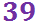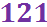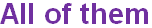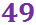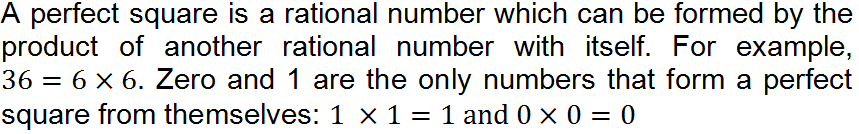Question 2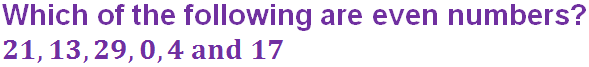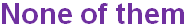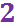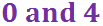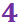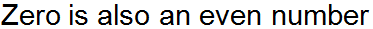Question 3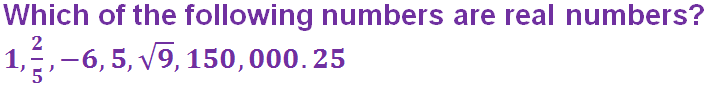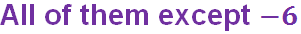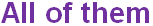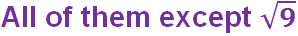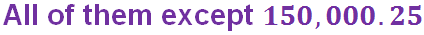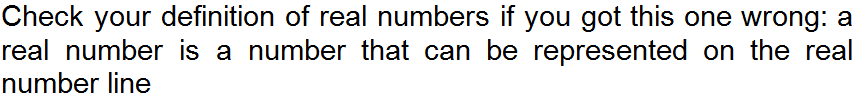Question 4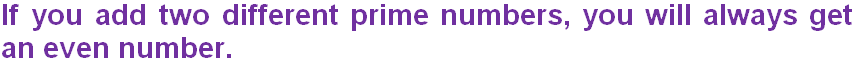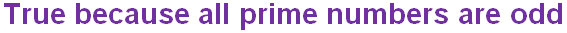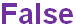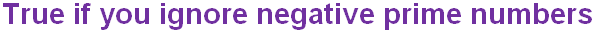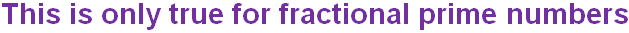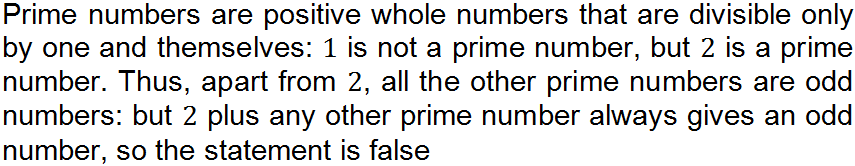Question 5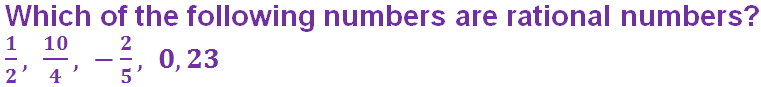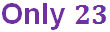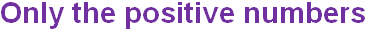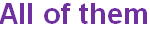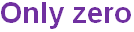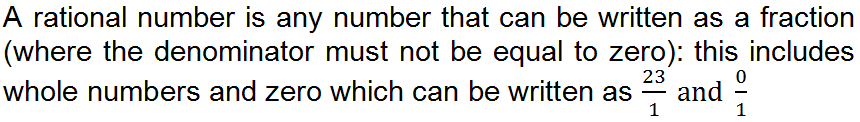Question 6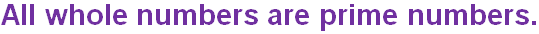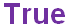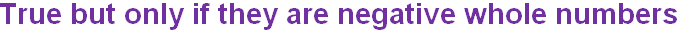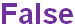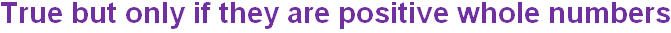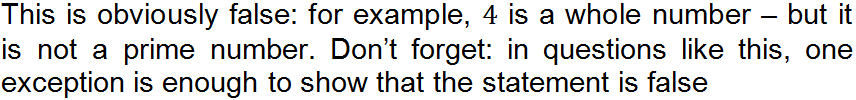Question 7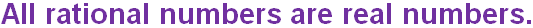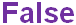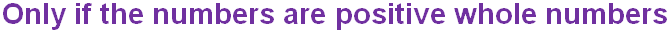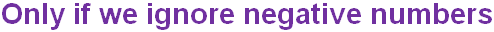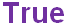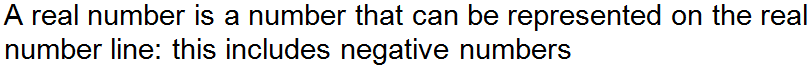Question 8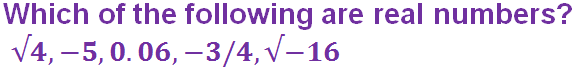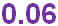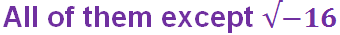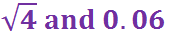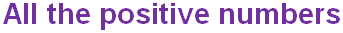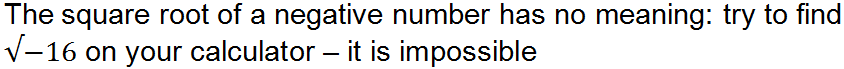Question 9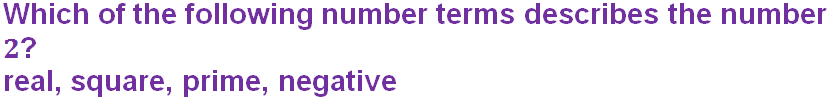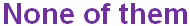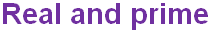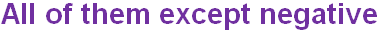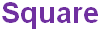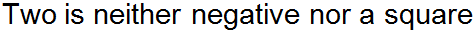Question 10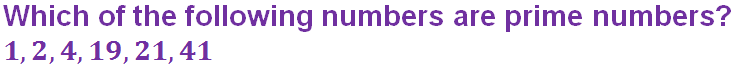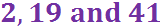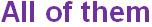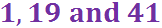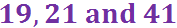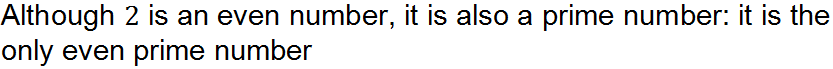Author:  Frank Evans